# [3.NF.1-1.0] What is a fraction - Common Core Standard

##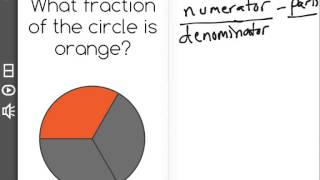By Front Row

Understand a fraction 1/b as the quantity formed by 1 part when a whole is partitioned into b equal parts# [3.NF.1-1.0] What is a fraction - Common Core Standard

##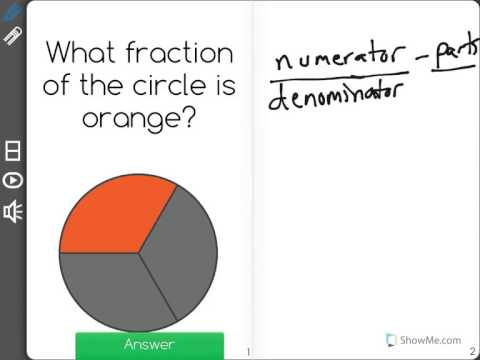By Freckle education

Understand a fraction 1/b as the quantity formed by 1 part when a whole is partitioned into b equal parts# Fractions of a Set

##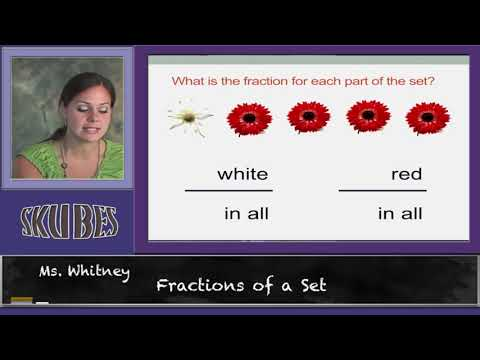By Skubes ed

Understand a fraction 1/b as the quantity formed by 1 part when a whole is partitioned into b equal parts; understand a fraction a/b as the quantity formed by a parts of size 1/b.# Understanding fractions (3.NF.1)

##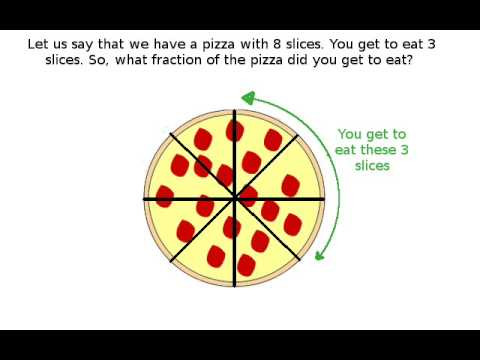By Far East Software

Understand a fraction 1/b as the quantity formed by 1 part when a whole is partitioned into b equal parts; understand a fraction a/b as the quantity formed by a parts of size 1/b.# [3.NF.2a-2.0] Unit Fractions on Number Line - Common Core Standard

##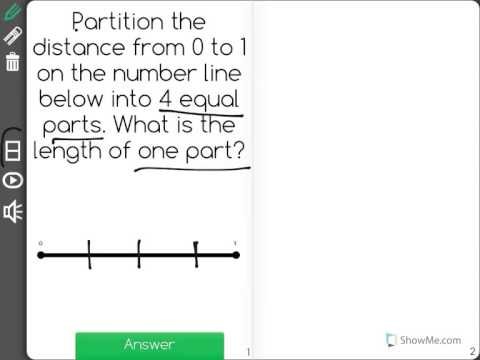By Freckle education

Represent a fraction 1/b on a number line diagram by defining the interval from 0 to 1 as the whole and partitioning it into b equal parts, recognizing that each part has size 1/b# 3.NF.2 - 3.MD.4

##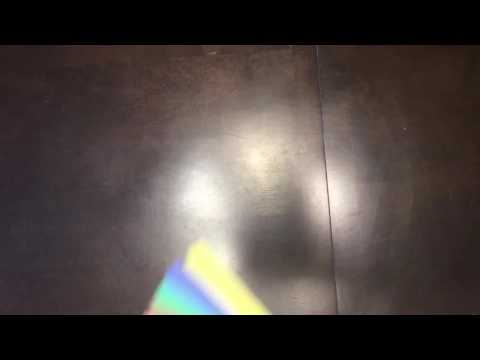By Simply math

Represent a fraction 1/b on a number line diagram by defining the interval from 0 to 1 as the whole and partitioning it into b equal parts. Recognize that each part has size 1/b and that the endpoint of the part based at 0 locates the number 1/b on the number line.# Fractions 4th Grade

##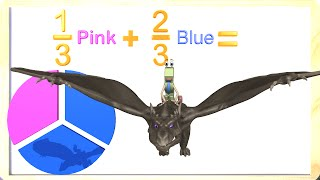By Math & Learning Videos 4 Kids

This video teaches fractions to 3rd and 4th graders. Kids will learn about fractions and how they get divided equally. A fraction represents a part of a whole. Kids will enjoy learning in this fun video with the Number One and his pet Dragon.# Types Of Fractions - Review | Maths For Grade 5 | Periwinkle

##By Lumos Learning

Types Of Fractions - Review | Maths For Grade 5 | Periwinkle# Math Antics - Adding Mixed Numbers

##By# Review of Fraction Concepts - 5th Grade Math

##By Lumos Learning

Get the full course at: http://www.MathTutorDVD.com​ In this lesson, you will learn what a fraction is and why it is important. We will begin by introducing the concept of a fraction as part / whole. The student will learn to draw fractions graphically to understand what the fraction represents. View more lessons at ScienceAndMath.com# Algebra Basics: What Is Algebra? - Math Antics

##By Lumos Learning

This video gives an overview of Algebra and introduces the concepts of unknown values and variables. It also explains that multiplication is implicit in Algebra.# 4th Grade Visualizing with Fractions

##By Lumos Learning

4th grade math lesson on visualizing with fractions.# 2nd Grade Math Compilation

##By

2nd Grade math is fun with these learning videos! Each lesson can help students with word problems, math games or help prepare for a test! Great for a review of 2nd grade or for an introduction!# Math Antics - Polygons

##By# ALL OF GRADE 9 MATH IN 60 MINUTES!!! (exam review part 2)

##By Lumos Learning

Here is a great exam review video reviewing all of the main concepts you would have learned in the MPM1D grade 9 academic math course. The video is divided in to 3 parts. This is part 2: Linear Relations. In this video you will review everything there is to know about y=mx+b, scatterplots, and distance time graphs.# Math Antics - Long Division with 2-Digit Divisors

##By# Math Antics - Telling Time

##By Lumos Learning

Learn More at mathantics.com Visit http://www.mathantics.com​ for more Free math videos and additional subscription based content!# How to escape education's death valley | Sir Ken Robinson

##By Lumos Learning

Sir Ken Robinson outlines 3 principles crucial for the human mind to flourish -- and how current education culture works against them. In a funny, stirring talk he tells us how to get out of the educational "death valley" we now face, and how to nurture our youngest generations with a climate of possibility# Word Study in Action: Vocabulary Development

##By Knatim

In this video, the teacher presents an open word sort.# Word Study in Action: Vocabulary Development

##By Knatim

In this video, the teacher presents an open word sort.